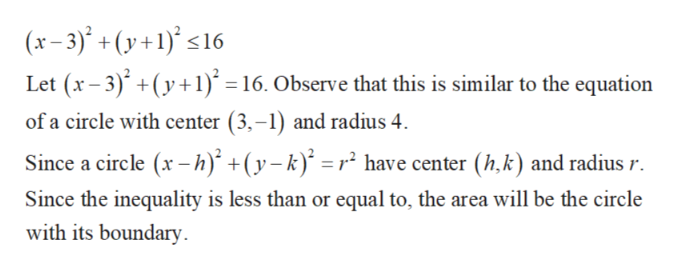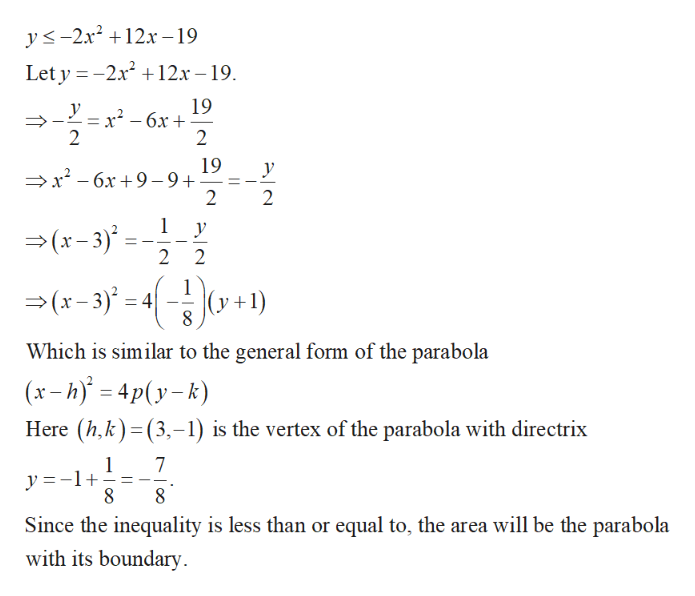# Can you please graph the following system of equations:?(x-3)^2 + (y+1)^2 </- 16 (that's less than or equal to 16)y</- -2x^2+12x-19  (that is also less than or equal to and negative 2x squared)

Question
58 views

Can you please graph the following system of equations:?

(x-3)^2 + (y+1)^2 </- 16 (that's less than or equal to 16)

y</- -2x^2+12x-19  (that is also less than or equal to and negative 2x squared)

check_circle

Step 1

Plot both the inequalities and find out the common area. Consider the first inequality and make it as an equation and plot the inequality as follows.help_outlineImage Transcriptionclose(x-3)+(y+1)s16 Let (x-3)y1) = 16. Observe that this is similar to the equation of a circle with center (3,-1) and radius 4 Since a circle (x-h) +(y-k) =r have center (h,k) and radius r Since the inequality is less than or equal to, the area will be the circle with its boundary fullscreen
Step 2

Plot the first inequality as follows.

Step 3

Consider the second inequality and make it as an e...help_outlineImage Transcriptionclosey-2x212x -19 Let y -2x212x -19 _ 19 x2 6x 2 У 2 19 x-6x +9-9+ 2 У 2 1 у x-3) 2 2 (x-3)4 (y +1) 8 Which is similar to the general form of the parabola (x-h)4p(y-k) Here (h,k) (3,-1) is the vertex of the parabola with directrix _ 1 y =-1+ 8 7 8 Since the inequality is less than or equal to, the area will be the parabola with its boundary fullscreen

### Want to see the full answer?

See Solution

#### Want to see this answer and more?

Solutions are written by subject experts who are available 24/7. Questions are typically answered within 1 hour.*

See Solution
*Response times may vary by subject and question.
Tagged in

### Algebra